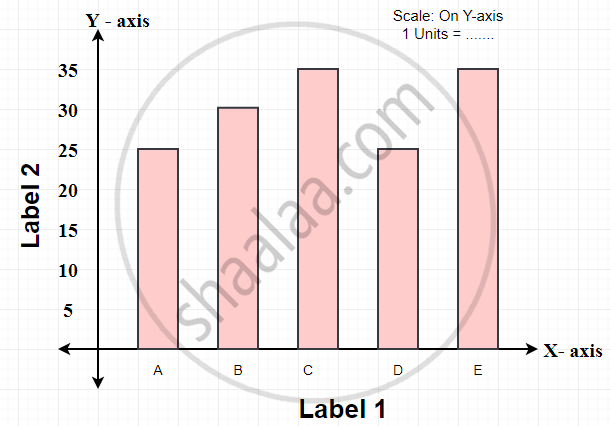# Concept of Bar Graph

#### definition

Bar graph: A bar graph is a representation of numbers using bars of uniform width and the lengths of the bars depend upon the frequency and the scale you have chosen.

# Graphical Representation of Data:

• Data is represented graphically to give a clear idea of what it represents.

• It is well said that one picture is better than a thousand words.

• Usually, comparisons among the individual items are best shown by means of graphs.

• The representation then becomes easier to understand than the actual data.

## 1. Bar Graph:

• A bar graph is used to show a comparison among categories.

• A bar graph is a representation of numbers using bars of uniform width and the lengths of the bars depend upon the frequency and the scale you have chosen.

• A bar chart or bar graph is a chart or graph that presents categorical data with rectangular bars with heights or lengths proportional to the values that they represent.

## Choosing a Scale:

• A bar graph is a representation of numbers using bars of uniform width and the lengths of the bars depend upon the frequency and the scale you have chosen.

• Large numbers cannot be represented in a bar graph, so the scaling factor is used to reduce or scale down large numbers.

## Parts of a Bar Graph:

• Title: The title explains what the graph is about.

• Scale: The scale of a bar graph is the range of values presented along either the horizontal or vertical axis. A bar graph is a representation of numbers using bars of uniform width and the lengths of the bars depend upon the frequency and the scale you have chosen. Large numbers cannot be represented in a bar graph, so the scaling factor is used to reduce or scale down large numbers.

• Interval: Interval is the smallest quantity between two tick marks along an axis.

• Labels: Both the side and the bottom of the bar graph have a label that tells what kind of data is shown. X-axis describes what each data point on the line represents and the y-axis shows the numeric value for each point on the line.

• Bars: The bar measures the data number.

• Key: Explains any additional information included in the graph. Many times, this will show different colors or symbols used to represent different categories. It is needed only when data about more than one category are shown in the graph.

• Data values: Data values are the actual numbers for each data point.If you would like to contribute notes or other learning material, please submit them using the button below.

### Shaalaa.com

Selecting Right Scale [00:15:43]
S
0%